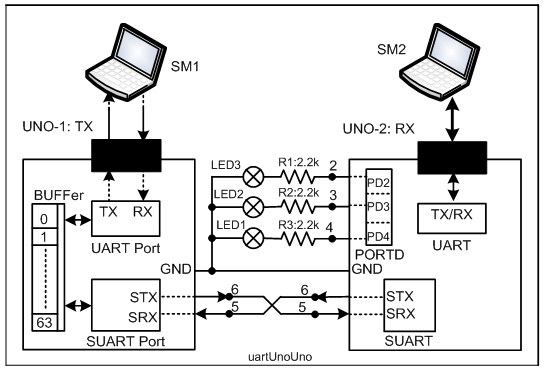# Master and slave code for temperature sensor TMP36

Hi I want to build the master and slaver arduino connected via UART port. In my master code I want it to be able to tell the temperature every time I drag the temperature bar and send out the message: normal, alert, and danger. After the message was sent out the slaver board will use it as the input to turn on individual LED: green, yellow, and red
I was able to get the mastercode to run perfectly fine. However, there was something wrong with my slaver code, it couldn't change anylight other than green. I tried to troubleshoot the issue, but I couldn't understand why, maybe there was sth wrong during the data transfer
This is my master code

char chArray;
int tmp36=A0;
int allowableTemp = 0;
double temp;
double tempC;
void setup()
{
Serial.begin(9600);
pinMode(tmp36, INPUT);
}
void loop()
{
allowableTemp = 30;
temp = temp / 1024; //* find percentage of input reading
temp = temp * 5; //* multiply by 5V to get voltage
temp = temp - 0.5; //* Subtract the offset
tempC = temp * 100; //* Convert to degree

if (tempC < allowableTemp) //temp below 30
{
}
else if (tempC >= allowableTemp && tempC < allowableTemp + 40) //temp between 30 and 70
{
}

else //temp above 70
{
}
}

Slaver code
char roomtemp ;
int LED1=4;
int LED2=3;
int LED3=2;
void setup ()
{
Serial.begin(9600);
pinMode(LED1, OUTPUT);
pinMode(LED2, OUTPUT);
pinMode(LED3, OUTPUT);
}
void loop ()
{
Serial.println(roomtemp);
if ("normal"){
digitalWrite(LED1,HIGH);
delay(1000);
digitalWrite(LED1,LOW);
delay(1000);
}
digitalWrite(LED2,HIGH);
delay(1000);
digitalWrite(LED2,LOW);
delay(1000);
}
else
{
digitalWrite(LED3,HIGH);
delay(1000);
digitalWrite(LED3,LOW);
delay(1000);
}
}

That term is always true (it's a character pointer which is never 0). I guess you're looking for something like strncmp().

1 Like

Yes but I don't know how it works, can you tell me a bit more about that command ? can I use it with if condition?

1. Connect two Arduinos as per following diagram (Fig-1) using SUART Port (Software UART Port). Also, connect the LEDs as shown.Figure-1:

2. Upload the following sketch (your one with slight modifications) in UNO-1.

``````#include<SoftwareSerial.h>
SoftwareSerial(5, 6);  //DPin-5 = SRX, DPin-6 = STX

char chArray;
int tmp36=A0;
int allowableTemp = 0;
double temp;
double tempC;

void setup()
{
Serial.begin(9600);
SUART.begin(9600);
pinMode(tmp36, INPUT);
}

void loop()
{
allowableTemp = 30;
temp = temp / 1024; //* find percentage of input reading
temp = temp * 5; //* multiply by 5V to get voltage
temp = temp - 0.5; //* Subtract the offset
tempC = temp * 100; //* Convert to degree

if (tempC < allowableTemp) //temp below 30
{
SUART.println(chArray);       //send message to Slave (UNO-2)
}
else if (tempC >= allowableTemp && tempC < allowableTemp + 40) //temp between 30 and 70
{
SUART.println(chArray);       //send message to Slave (UNO-2)
}
else //temp above 70
{
SUART.println(chArray);       //send message to Slave (UNO-2)
}
}
``````

3. Upload the following sketch (your one with slight modification) in Slave (UNO-2).

``````#include<SoftwareSerial.h>
SoftwareSerial(5, 6);  //DPin-5 = SRX, DPin-6 = STX
char roomtemp ;
int LED1=4;
int LED2=3;
int LED3=2;
byte m;

void setup ()
{
Serial.begin(9600);
SUART.begin(9600);
pinMode(LED1, OUTPUT);
pinMode(LED2, OUTPUT);
}

void loop()
{
while(SUART.available() >0)
{
}
roomtemp[m] = '\0';

if(strcmp(roomtemp, "normal") == 0)
{
digitalWrite(LED1, HIGH);
delay(1000);
digitalWrite(LED1, LOW);
delay(1000);
}

{
digitalWrite(LED2, HIGH);
delay(1000);
digitalWrite(LED2, LOW);
delay(1000);
}

if(strcmp(roomtemp, "normal") == 0)
{
digitalWrite(LED1, HIGH);
delay(1000);
digitalWrite(LED1, LOW);
delay(1000);
}
}
``````
1 Like

@GolamMostafa
Hi, I couldn't find SUART port on my arduino, is it only appear on the software

You don't need to worry about it. It is automatically created when you include the following lines (at the places you see in the sketch) in the sketch:

``````#include<SoftwareSerial.h>
SoftwareSerial SUART(5, 6); //DPin-5 = SRX, DPin-6 = STX
SUART.begin(9660);
``````

Have you tested the sketches of Post#4? Are they working?

1 Like

Yes, it works thank you so much

This topic was automatically closed 120 days after the last reply. New replies are no longer allowed.